Latest SSC jobs   »   Government Jobs   »   Venn Diagrams

# Venn Diagram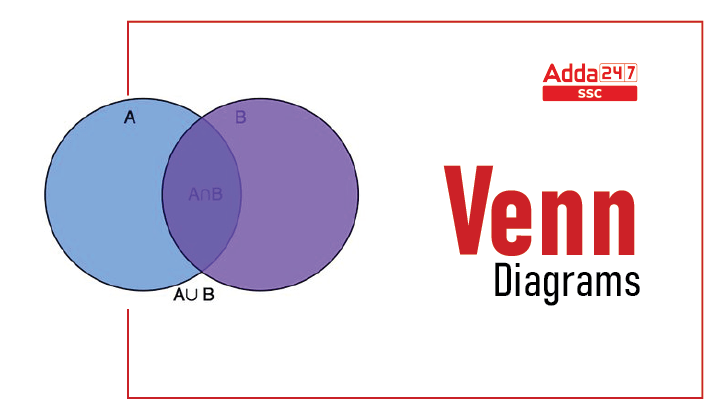## Venn Diagrams

Venn diagrams are the pictorial representation of elements that use circles or any other shapes to show the relationship between given elements. Circles that overlap have a commonality while circles that do not overlap do not share those aspects. Venn diagrams help to visually represent the similarities and variations between two concepts. Venn diagrams also named Set diagrams or Logic diagrams, are widely used in mathematics, statistics, logic, teaching, linguistics, computer science, and business to show the data in pictorial form. We are going to discuss here Venn diagrams examples with questions in detail.

## Venn Diagrams Reasoning

Venn diagram is an important topic in the general intelligence or reasoning section which is asked in almost all the exams. The Venn diagram in reasoning is used to represent the data or concept through diagrams or pictures. We are going to discuss the Venn Diagrams Questions and Examples in this article. So refer to this article to get all the details of Venn diagrams and their related concepts.

## Types of Venn Diagrams

Generally, Venn diagrams are of three types namely two-element Venn diagram, three-element Venn diagram, and four elements Venn diagram depending on the given condition. You can understand all the types through the Venn diagrams questions given in the article.

## Venn Diagrams Symbols

Venn diagrams symbols are used to represent the relationship between given elements. The Venn diagrams symbols with examples are discussed below.

• Union (∪): It represents the union of all the sets – i.e., the universe of all elements within X and Y sets. The union of the set is given here.
• Intersection (∩): Intersection represents all elements shared or common within the selected sets or groups. It represents the shared or common elements (in the middle) within the sets X and Y.
• Complement (XC): Complement represents whatever is not represented in a particular set; in this case, everything not in set X. An equation to illustrate the complement of X is XC = U/A, where U illustrates a given universe of elements. The diagram below shows the absolute complement of X in U.

## Venn Diagrams Formulas

Venn diagrams formulas are used to solve the problems based on Venn diagrams easily. The Venn diagrams formulas for 2 and 3 sets are discussed here.

• n ( A ∪ B) = n(A ) + n ( B ) – n ( A∩ B)
• n (A ∪ B ∪ C) = n(A ) + n ( B ) + n (C) – n ( A ∩ B) – n ( B ∩ C) – n ( C ∩ A) + n (A ∩ B ∩ C)
• And so on, where n(A) = number of elements in set A.

## Venn Diagrams for Sets

A set is a collection of things or elements shown by the pictures. Venn diagrams for sets are given here with examples. Refer to these questions to understand the topic in detail.

## Venn Diagrams Questions

Venn diagrams questions require practice and logical ability to solve them easily. We are going to discuss here some important Venn diagram questions for a better understanding of concepts. So practice these questions for your upcoming exams.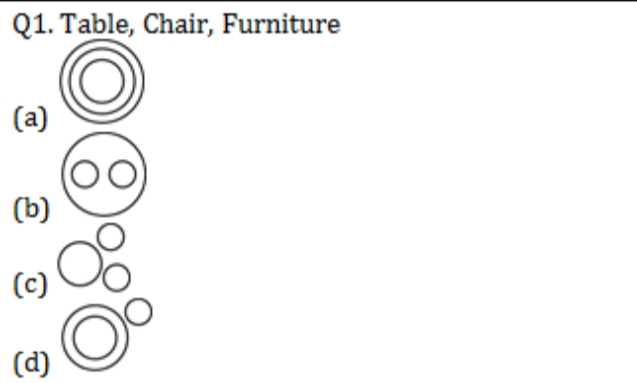Solution: Ans (b) The pictorial representation of the Table, Chair, and Furniture is shown here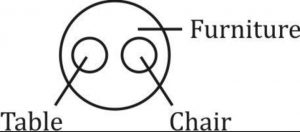The table and Chair are entirely different. But, both are items of furniture
Que.2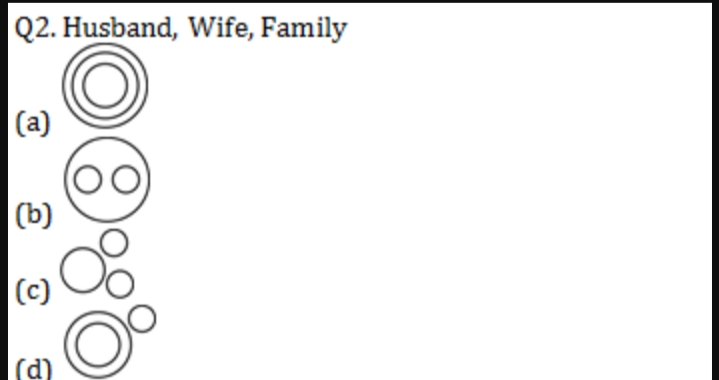Solution: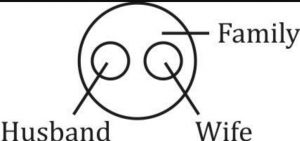Husband and Wife are entirely different. But, both are parts of a family.
Que.3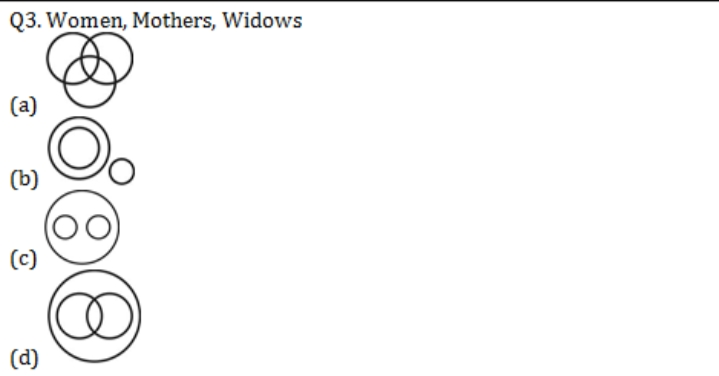Solution: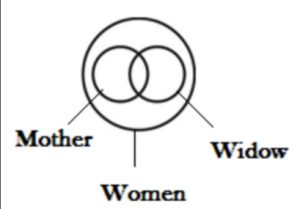All mothers and all widows are women. Some mothers can be a widow.
Que.4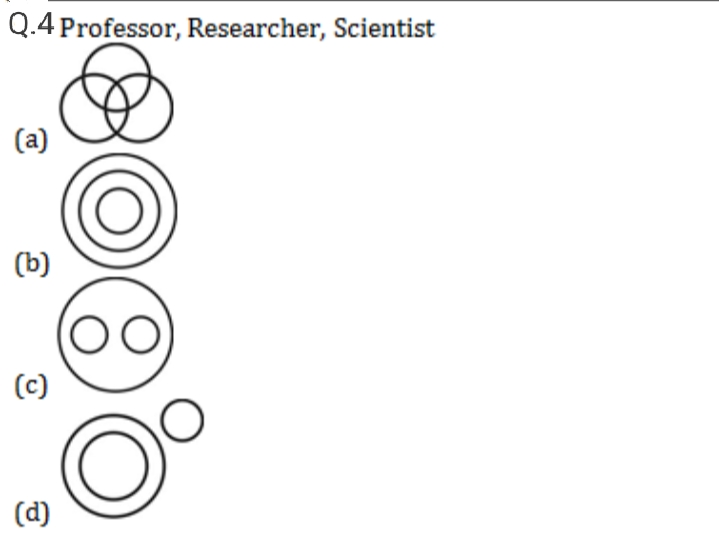Solution: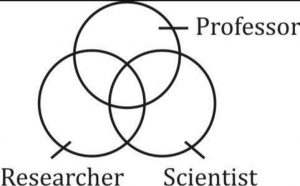Some professors may be scientists or researchers. Some scientists may be researchers.
Que.5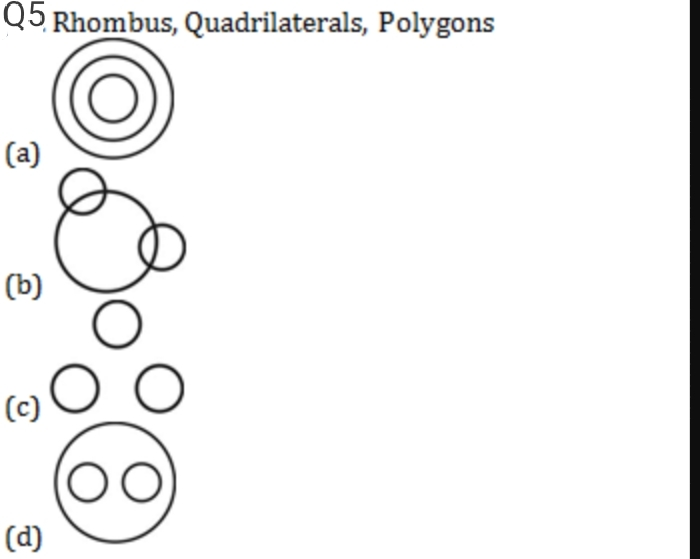Solution: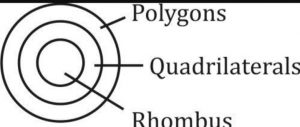Que.6 In the following questions, answers are to be based on the diagrams given below, where the triangle represents doctors, the circle represents players and the rectangle represents artists.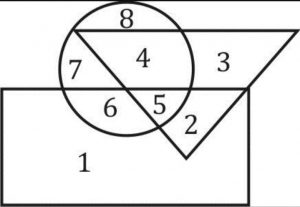I. Which numbered space in the diagram represents doctors who are also players and artists?
(a) 2
(b) 3
(c) 4
(d) 5
Ans. (d)
Sol. The required region is the one that is common to the triangle, circle, and rectangle i.e. 5.
II. Which number represents artists who are also players only?
(a) 4
(b) 6
(c) 7
(d) 8
Ans. (b)
Sol. The required region is the one that is common to the rectangle and the circle but lies outside the triangle i.e. 6.
III. Which number represents artists who are neither players nor doctors?
(a) 1
(b) 2
(c) 3
(d) 4
Ans. (a)
Sol. The required region is the one that lies inside the rectangle but outside the circle and the triangle i.e. 1.

## Venn Diagrams – FAQs

Que.1 Define Venn Diagram?
Ans – A Venn diagram is a pictorial representation of elements followed by given conditions.
Que.2 How many types of Venn diagrams are there?
Ans – Venn diagrams are of three types namely two elements, three elements, and four elements Venn diagrams.